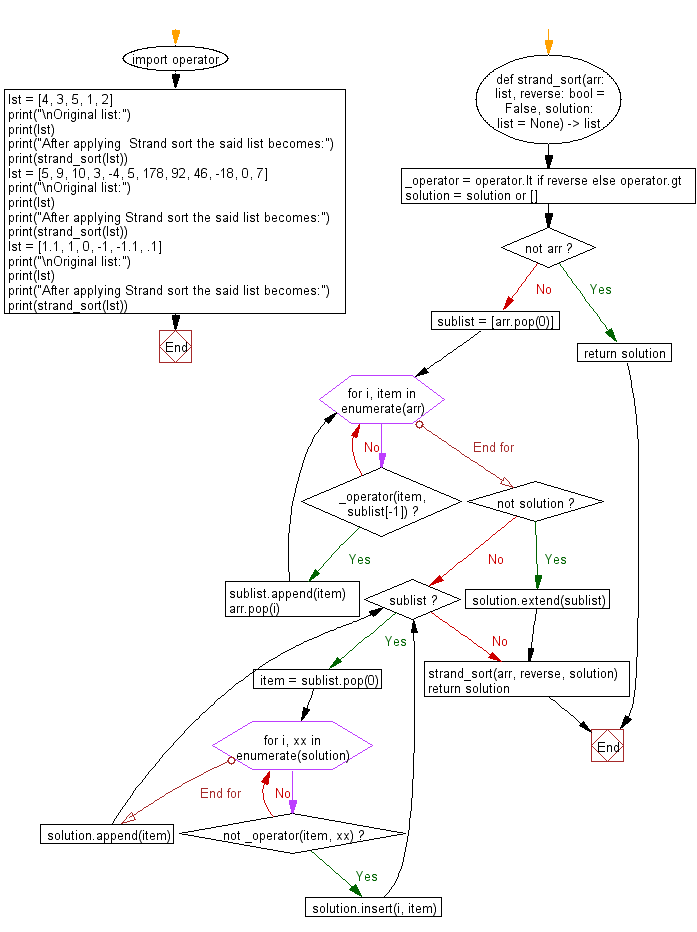﻿ Python: Sort unsorted numbers using Strand sort - w3resource# Python: Sort unsorted numbers using Strand sort

## Python Search and Sorting : Exercise-26 with Solution

Write a Python program to sort unsorted numbers using Strand sort.

Strand sort is a recursive sorting algorithm that sorts items of a list into increasing order. It has O(n2) worst time complexity which occurs when the input list is reverse sorted. It has a best case time complexity of O(n) which occurs when the input is a list that is already sorted. Strand sort is not in-place as its space complexity is O(n).

Sample Solution:

Python Code:

``````#Ref:https://bit.ly/3qW9FIX
import operator
def strand_sort(arr: list, reverse: bool = False, solution: list = None) -> list:
_operator = operator.lt if reverse else operator.gt
solution = solution or []
if not arr:
return solution
sublist = [arr.pop(0)]
for i, item in enumerate(arr):
if _operator(item, sublist[-1]):
sublist.append(item)
arr.pop(i)

#  merging sublist into solution list
if not solution:
solution.extend(sublist)
else:
while sublist:
item = sublist.pop(0)
for i, xx in enumerate(solution):
if not _operator(item, xx):
solution.insert(i, item)
break
else:
solution.append(item)

strand_sort(arr, reverse, solution)
return solution
lst = [4, 3, 5, 1, 2]
print("\nOriginal list:")
print(lst)
print("After applying  Strand sort the said list becomes:")
print(strand_sort(lst))
lst = [5, 9, 10, 3, -4, 5, 178, 92, 46, -18, 0, 7]
print("\nOriginal list:")
print(lst)
print("After applying Strand sort the said list becomes:")
print(strand_sort(lst))
lst = [1.1, 1, 0, -1, -1.1, .1]
print("\nOriginal list:")
print(lst)
print("After applying Strand sort the said list becomes:")
print(strand_sort(lst))
```
```

Sample Output:

```Original list:
[4, 3, 5, 1, 2]
After applying  Strand sort the said list becomes:
[1, 2, 3, 4, 5]

Original list:
[5, 9, 10, 3, -4, 5, 178, 92, 46, -18, 0, 7]
After applying Strand sort the said list becomes:
[-18, -4, 0, 3, 5, 5, 7, 9, 10, 46, 92, 178]

Original list:
[1.1, 1, 0, -1, -1.1, 0.1]
After applying Strand sort the said list becomes:
[-1.1, -1, 0, 0.1, 1, 1.1]
```

Flowchart:Python Code Editor:

What is the difficulty level of this exercise?

Test your Programming skills with w3resource's quiz.

﻿

## Python: Tips of the Day

Use Reversed() In for Loops:

```>>> tasks = ['laundry', 'picking up kids', 'gardening', 'cooking']